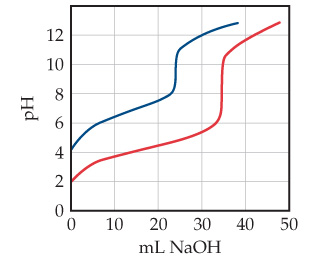# Problem: Equal volumes of two acids are titrated with 0.10 M NaOH resulting in the two titration curves shown in the following figureWhich curve corresponds to the more concentrated acid solution?

###### FREE Expert Solution

In this problem, we are asked to determine which of the two titration curves corresponds to the more concentrated acid solution.

Titration curves help us to understand the neutralization occurring between an acid and base in order to identify the equivalence point.

We answer the problem by examining the titration curve given:

83% (268 ratings)###### Problem Details

Equal volumes of two acids are titrated with 0.10 M NaOH resulting in the two titration curves shown in the following figureWhich curve corresponds to the more concentrated acid solution?